Courses

# Stoichiometry MCQ - 1 (Advance)

## 30 Questions MCQ Test Chemistry for JEE Advanced | Stoichiometry MCQ - 1 (Advance)

Description
This mock test of Stoichiometry MCQ - 1 (Advance) for JEE helps you for every JEE entrance exam. This contains 30 Multiple Choice Questions for JEE Stoichiometry MCQ - 1 (Advance) (mcq) to study with solutions a complete question bank. The solved questions answers in this Stoichiometry MCQ - 1 (Advance) quiz give you a good mix of easy questions and tough questions. JEE students definitely take this Stoichiometry MCQ - 1 (Advance) exercise for a better result in the exam. You can find other Stoichiometry MCQ - 1 (Advance) extra questions, long questions & short questions for JEE on EduRev as well by searching above.
QUESTION: 1

### Match the coloumn: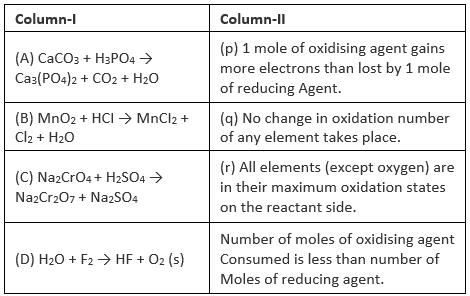Solution:
*Multiple options can be correct
QUESTION: 2

### Which of the following samples of reducing agents is /are chemically equivalent to 25 ml of 0.2 N KMnO4 to be reduced to Mn2+ and water.

Solution:
*Multiple options can be correct
QUESTION: 3

### There are two sample of CI having polarity 1M and 0.25 M. Fin d volume of these sample taken in order to prepare 0.75 M HCL solution. (Assume no water is used)

Solution: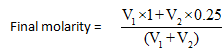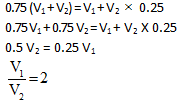*Multiple options can be correct
QUESTION: 4

Chloride of an element is given by the formula MCI, and it is 100% ionised in 0.01 M aqueous solution.Then

Solution:

MClx

In each mole of MClx there are x moles of Cl

⇒       [Cl-] = x * 0.01

conc. of [Mx+] = 0.01

*Multiple options can be correct
QUESTION: 5

If 100 ml of 1M H2SO4 solution is mixed with 100 ml of 98%(wlw) H2SO4 solution (d = 0.1 gmlml) then :

Solution: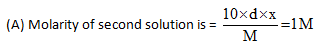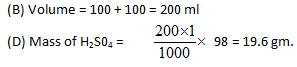*Multiple options can be correct
QUESTION: 6

3 moles of the gas C2H6is mixed with 60 gm of this gas and 2.4 x 1024 molecules of the gas is removed. The left over gas is combusted in the presence of excess oxygen then : (NA = 6 x 1023) (Density of water = 1 gm/ml)

Solution:

Moles of C2H6 = 3

moles of C2H6 mixed = 60/30 = 2

total mole of C2H6 = 5

moles removed =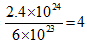moles of C2 H6 left =1

Now, C2 H6 + 7/2 O2 → 2CO2 + 3H2O

Clearly 3 moles of H2O or 54 gm H2O will be formed

volume of H2O = 54 ml

*Multiple options can be correct
QUESTION: 7

Which of the following contains the same number of molecules?

Solution:

For same number of molecules, number of moles should be same.

QUESTION: 8

For producing 4 gram moles of HNO3, how many grams of NO2 are required?

Solution:

3 gram mole of NO2 = 2 gram moles of HNO3.

*Multiple options can be correct
QUESTION: 9

Choose the correct statement :

Solution:

Use equivalent of oxidising agent = equivalents of reducing agent.

QUESTION: 10

632 g of sodium thiosulphate (Na2S2O3) reacts with copper sulphate to form cupric thiosulphate which is reduced by sodium thiosulphate to give cuprous compound which is dissolved in excess of sodium thiosulphate to form a complex compound sodium cuprothiosulphate ( Na4[Cu6(S2O3)5] ).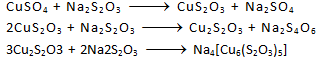Sodium cuprothiosulphaté

In this process, 0.2 mole of sodium cuprothiosulphate is formed. (O = 16, Na = 23, S = 32)

The average oxidation states of sulphur in Na2S2O3 and Na2S4O6 are respectively.

Solution:
QUESTION: 11

632 g of sodium thiosulphate (Na2S2O3) reacts with copper sulphate to form cupric thiosulphate which is reduced by sodium thiosulphate to give cuprous compound which is dissolved in excess of sodium thiosulphate to form a complex compound sodium cuprothiosulphate ( Na4[Cu6(S2O3)5] ).Sodium cuprothiosulphaté

In this process, 0.2 mole of sodium cuprothiosulphate is formed. (O = 16, Na = 23, S = 32)

Q.

Moles of sodium thiosulphate reacted and unreacted after the reaction are respecticvely.

Solution:

Moles of Na2S2O3 used = 0.4 + 0.6 + 1.2 = 2.2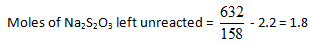QUESTION: 12

632 g of sodium thiosulphate (Na2S2O3) reacts with copper sulphate to form cupric thiosulphate which is reduced by sodium thiosulphate to give cuprous compound which is dissolved in excess of sodium thiosulphate to form a complex compound sodium cuprothiosulphate ( Na4[Cu6(S2O3)5] ).Sodium cuprothiosulphaté

In this process, 0.2 mole of sodium cuprothiosulphate is formed. (O = 16, Na = 23, S = 32)

Q.

If instead of given amount of sodium thiosulphate, 2 moles of sodium thiosulphate along with 3 moles of CuSO4were taken initially. Then moles of sodium cuprothiosulphate formed is

Solution:

No moles of Na2S2O3 would remain in step

(i) so further reaction will stop because Na2S2O3 is required in excess.

QUESTION: 13

We know that balancing of a chemical equation is entirely based on law of conservation of mass. However the concept of Principle of Atom Conservation (POAC) can also be related to law of conservation of mass in a chemical reaction. So, POAC can also act as a technique for balancing a chemical equation. For example, for a reaction

2AB3 → AB + C2

0n applying POAC for, B & C and relating the 3 equations, we get :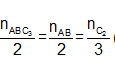( nx :  number of
moles of X) Thus, the coefficients of ABC3 , AB & C2 in the balanced chemical equation will be 2,2 & 3 respectively and the balanced chemical equation can be represented as

ABC3 → AB + 3C2

Now answer the following questions :

Q.

Which of the following relation is correct regarding the numerical coefficients p, q , r in the balanced chemical equation :

pA + qB2 → rA2B5

Solution: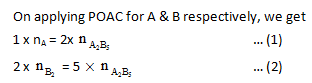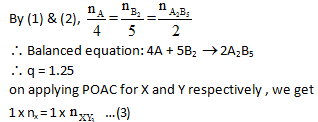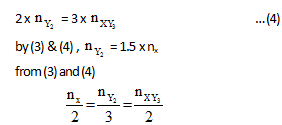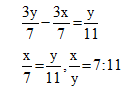QUESTION: 14

We know that balancing of a chemical equation is entirely based on law of conservation of mass. However the concept of Principle of Atom Conservation (POAC) can also be related to law of conservation of mass in a chemical reaction. So, POAC can also act as a technique for balancing a chemical equation. For example, for a reaction

2AB3 → AB + C2

0n applying POAC for, B & C and relating the 3 equations, we get :( nx :  number of
moles of X) Thus, the coefficients of ABC3 , AB & C2 in the balanced chemical equation will be 2,2 & 3 respectively and the balanced chemical equation can be represented as

ABC3 → AB + 3C2

Now answer the following questions :

Q.

If the weight ratio of C and 02 present is 1 : 2 and both of reactants completely consume and form C0 and C02 and we will obtain a gasous mixture of C0 and CO2. What would be the weight ratio of C0 and C02 in mixture.

Solution:QUESTION: 15

We know that balancing of a chemical equation is entirely based on law of conservation of mass. However the concept of Principle of Atom Conservation (POAC) can also be related to law of conservation of mass in a chemical reaction. So, POAC can also act as a technique for balancing a chemical equation. For example, for a reaction

ABC3 → AB + C2

0n applying POAC for, B & C and relating the 3 equations, we get :( nx :  number of
moles of X) Thus, the coefficients of ABC3 , AB & C2 in the balanced chemical equation will be 2,2 & 3 respectively and the balanced chemical equation can be represented as

2ABC3 → 2AB + 3C2

Now answer the following questions :

Q.

If the atomic masses of X and Y are 10 & 30 respectivetly, then the mass of XY3 formed when 120g of Y2 reacts completely with X is : Reaction X + Y2 ¾¾® XY3

Solution:QUESTION: 16

Oleum is considered as a solution of S03 in H2S04 , which is obtained by passing 803 in solution of H2SO4. When 100 g sample of oleum is diluted with desired weight of H20 then the total mass of H2SO4 obtained after dilution is known as % labelling in oleum.
For example, a oleum bottle labelled as '109% H2SO4 means the 109g total mass of pure H2SO4 will be formed when 100 g of oleum is diluted by 9 g of Hp which combines with all the free 803 to form H2SO4 as

S03 + H20 → + H2SO4.

Q.

What is the % of free S03 in an oleum that is labelled as '104.5% H2SO4’?

Solution:

SO3 + H2O →H2SO4. ;

18 g water combines with 80 g SO3

∴ 4.5 g of H2O combines with 20 g of SO3

∴ 100 g of oleum contains 20 g of SO3 or 20% free SO3

QUESTION: 17

Oleum is considered as a solution of S03 in H2S04 , which is obtained by passing 803 in solution of H2SO4. When 100 g sample of oleum is diluted with desired weight of H20 then the total mass of H2SO4 obtained after dilution is known as % labelling in oleum.
For example, a oleum bottle labelled as '109% H2SO4 means the 109g total mass of pure H2SO4 will be formed when 100 g of oleum is diluted by 9 g of Hp which combines with all the free 803 to form H2SO4 as

S03 + H20 → + H2SO4.

Q.

If excess water is added into a 100 g bottle sample labelled as '' 112% H2SO4 '' and is reacted with 5.3 g Na2CO3, then find the volume of C02 evolved at 1 atm pressure and 300 k temperature after the completion of the reaction : [R = 0.0821 L atom mol–1 K1]

H2SO4 + Na2CO3 → Na2SO4 + H20 + CO2

Solution:

H2SO4 + Na2CO3 → Na2SO4 + H2O + CO2

Moles of CO2 formed = moles of Na2CO3 reacted (it is limiting reagent)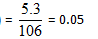volume of CO2 formed at 1 atm pressure and 300 K = 0.05 * 24.63 = 1.23 L.

QUESTION: 18

Oleum is considered as a solution of S03 in H2S04 , which is obtained by passing 803 in solution of H2SO4. When 100 g sample of oleum is diluted with desired weight of H20 then the total mass of H2SO4 obtained after dilution is known as % labelling in oleum.
For example, a oleum bottle labelled as '109% H2SO4 means the 109g total mass of pure H2SO4 will be formed when 100 g of oleum is diluted by 9 g of Hp which combines with all the free 803 to form H2SO4 as

S03 + H20 → + H2SO4.

Q.

1 g of oleum sample is diluted with water.The solution required 54 ml o f 0.4 N NaOH for  compete neutralization.The % of free S03 in the sample is :

Solution:

Equivalent of H2SO4 + equivalent of SO3 = equivalent of NaOH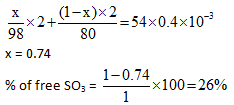QUESTION: 19

Volumetric analysis is based on the principle of equivalence a that minloces lead in the ratio of their equivalents. At the equivalence point of the reaction, involving the reactahts A and B : Number of gram equivalents of A = Number of gram equivalents of B..
If VA ml of solution A having normality NA react just completely with VB ml  of solution B having normalityNB then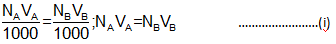Equation(1), called normality equation is very useful in numerical of volumetric analysis. Equivalent masses of different substances :

Basically the equivalent mass of a substance is defined as the pads by seam

ctituhich combine with or displace 1.0078 parts (≈1part) by mass of hydrogen, 8 parts by mansetaisiosaid 35.5 parts by mass of chlorine.

Mass of a substance expressed in gram equal to its equivalent man is' gam equivalent mass. Equivalent mass of a substance is not constant but depends upon the maw i malice the substance participates.

Equivalent mass of an acid in acid-base reaction is its mass in grannehidicodoinisooleaf replaceable Fi ions (= 1.0078 g≈1g).0n the other hand, equivalent mass of a bagels istolonellfacontairis 1 mole of replaceable 0H ions. 1 g equivalent mass each of an acid and base an ionclinegins saint and 1 mole of water ( = 18 g).

Equivalent mass of an oxidising agent is its mass which gains 1 mole at ellecismitcan be obtained by dividing the molecular mass or formula mass by the total decrease in aididditaiiilberef one or more elements per molecule.

0n the other hand, equivalent mass of a reducing agent is the mass of the substance which loses 1 mole of electorns. It can be calculated by dividing the molecular or formula  mass of the subtance by the total increase in oxidation number of one or more elements per molecular or formula mass.

Q.

When 5.00 g of a metal is strongly heated, 9.44 g of its oxide is obtained. The equivalent mass of the metal is

Solution:

Mass of oxygen in oxide = 9.44 - 5 = 4.44

Eq. wt. of metal = mass of metal which combine with 8 gm of oxygen

= 5/4.44*8 8 = 9.00 gm

QUESTION: 20

Volumetric analysis is based on the principle of equivalence a that minloces lead in the ratio of their equivalents. At the equivalence point of the reaction, involving the reactahts A and B : Number of gram equivalents of A = Number of gram equivalents of B..
If VA ml of solution A having normality NA react just completely with VB ml  of solution B having normalityNB thenEquation(1), called normality equation is very useful in numerical of volumetric analysis. Equivalent masses of different substances :

Basically the equivalent mass of a substance is defined as the pads by seam ctituhich combine with or displace 1.0078 parts (≈1part) by mass of hydrogen, 8 parts by mansetaisiosaid 35.5 parts by mass of chlorine.

Mass of a substance expressed in gram equal to its equivalent man is' gam equivalent mass. Equivalent mass of a substance is not constant but depends upon the maw i malice the substance participates.

Equivalent mass of an acid in acid-base reaction is its mass in grannehidicodoinisooleaf replaceable Fi ions (= 1.0078 g≈1g).0n the other hand, equivalent mass of a bagels istolonellfacontairis 1 mole of replaceable 0H ions. 1 g equivalent mass each of an acid and base an ionclinegins saint and 1 mole of water= 18 g). Equivalent mass of an oxidising agent is its mass which gains 1 mole at ellecismitcan be obtained by dividing the molecular mass or formula mass by the total decrease in aididditaiiilberef one or more elements per molecule.

0n the other hand, equivalent mass of a reducing agent is the mass of the substance which loses 1 mole of electorns. It can be calculated by dividing the molecular or formula  mass of the subtance by the total increase in oxidation number of one or more elements per molecular or formula mass.

Q.

How many gram of phosphoric acid (H3PO4) would be required to neutralize 58 g of magnesium hydroxide?

Solution: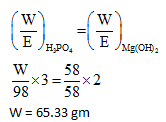QUESTION: 21

Volumetric analysis is based on the principle of equivalence a that minloces lead in the ratio of their equivalents. At the equivalence point of the reaction, involving the reactahts A and B : Number of gram equivalents of A = Number of gram equivalents of B..
If VA ml of solution A having normality NA react just completely with VB ml  of solution B having normalityNB thenEquation(1), called normality equation is very useful in numerical of volumetric analysis. Equivalent masses of different substances :

Basically the equivalent mass of a substance is defined as the pads by seam ctituhich combine with or displace 1.0078 parts (≈1part) by mass of hydrogen, 8 parts by mansetaisiosaid 35.5 parts by mass of chlorine.

Mass of a substance expressed in gram equal to its equivalent man is' gam equivalent mass. Equivalent mass of a substance is not constant but depends upon the maw i malice the substance participates.

Equivalent mass of an acid in acid-base reaction is its mass in grannehidicodoinisooleaf replaceable Fi ions (= 1.0078 g≈1g).0n the other hand, equivalent mass of a bagels istolonellfacontairis 1 mole of replaceable 0H ions. 1 g equivalent mass each of an acid and base an ionclinegins saint and 1 mole of water= 18 g). Equivalent mass of an oxidising agent is its mass which gains 1 mole at ellecismitcan be obtained by dividing the molecular mass or formula mass by the total decrease in aididditaiiilberef one or more elements per molecule.

0n the other hand, equivalent mass of a reducing agent is the mass of the substance which loses 1 mole of electorns. It can be calculated by dividing the molecular or formula  mass of the subtance by the total increase in oxidation number of one or more elements per molecular or formula mass.

Q.

Which of the following solutions, when mixed with 100 ml of 0.05 M NaOH, will give a neutral solution?

Solution:

Equilise the m.e. of NaOH by m.e. of acid.

QUESTION: 22

Volumetric analysis is based on the principle of equivalence a that minloces lead in the ratio of their equivalents. At the equivalence point of the reaction, involving the reactahts A and B : Number of gram equivalents of A = Number of gram equivalents of B..
If VA ml of solution A having normality NA react just completely with VB ml  of solution B having normalityNB thenEquation(1), called normality equation is very useful in numerical of volumetric analysis. Equivalent masses of different substances :

Basically the equivalent mass of a substance is defined as the pads by seam ctituhich combine with or displace 1.0078 parts (≈1part) by mass of hydrogen, 8 parts by mansetaisiosaid 35.5 parts by mass of chlorine.

Mass of a substance expressed in gram equal to its equivalent man is' gam equivalent mass. Equivalent mass of a substance is not constant but depends upon the maw i malice the substance participates.

Equivalent mass of an acid in acid-base reaction is its mass in grannehidicodoinisooleaf replaceable Fi ions (= 1.0078 g≈1g).0n the other hand, equivalent mass of a bagels istolonellfacontairis 1 mole of replaceable 0H ions. 1 g equivalent mass each of an acid and base an ionclinegins saint and 1 mole of water= 18 g). Equivalent mass of an oxidising agent is its mass which gains 1 mole at ellecismitcan be obtained by dividing the molecular mass or formula mass by the total decrease in aididditaiiilberef one or more elements per molecule.

0n the other hand, equivalent mass of a reducing agent is the mass of the substance which loses 1 mole of electorns. It can be calculated by dividing the molecular or formula  mass of the subtance by the total increase in oxidation number of one or more elements per molecular or formula mass.

Q.

100 ml of 0.1 M H3 P02 is titrated with 0.1 M NaOH. The volume of NaOH needed will be

Solution:

M.e. of H3PO2 = m.e. of NaOH

100 * 0.1 * 1 = V * 0.1

V = 100 ml

H3PO2 is monbasic acid

QUESTION: 23

Volumetric analysis is based on the principle of equivalence a that minloces lead in the ratio of their equivalents. At the equivalence point of the reaction, involving the reactahts A and B : Number of gram equivalents of A = Number of gram equivalents of B..
If VA ml of solution A having normality NA react just completely with VB ml  of solution B having normalityNB thenEquation(1), called normality equation is very useful in numerical of volumetric analysis. Equivalent masses of different substances :

Basically the equivalent mass of a substance is defined as the pads by seam ctituhich combine with or displace 1.0078 parts (≈1part) by mass of hydrogen, 8 parts by mansetaisiosaid 35.5 parts by mass of chlorine.

Mass of a substance expressed in gram equal to its equivalent man is' gam equivalent mass. Equivalent mass of a substance is not constant but depends upon the maw i malice the substance participates.

Equivalent mass of an acid in acid-base reaction is its mass in grannehidicodoinisooleaf replaceable Fi ions (= 1.0078 g≈1g).0n the other hand, equivalent mass of a bagels istolonellfacontairis 1 mole of replaceable 0H ions. 1 g equivalent mass each of an acid and base an ionclinegins saint and 1 mole of water= 18 g). Equivalent mass of an oxidising agent is its mass which gains 1 mole at ellecismitcan be obtained by dividing the molecular mass or formula mass by the total decrease in aididditaiiilberef one or more elements per molecule.

0n the other hand, equivalent mass of a reducing agent is the mass of the substance which loses 1 mole of electorns. It can be calculated by dividing the molecular or formula  mass of the subtance by the total increase in oxidation number of one or more elements per molecular or formula mass.

Q.

9.8 g of an acid on treatment with excess of an alkali forms salt along with 3.6 g of water. What is the equivalent mass of the acid?

Solution:

Number of moles of water produced = 0.2 moles

∴ 0.2 equivalent of acid should be reacted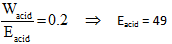QUESTION: 24

Molality : It is defined as the moles of the solute present in 1kg of the solvent. It is denoted by 'm'.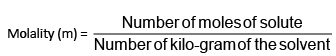Let WA grams of the solute of molecular mass mA be present in WB grams of the solvent, then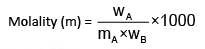Relation between mole fraction and Molality :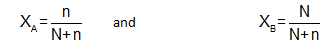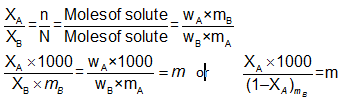Q.

If the ratio of the mole fraction of a solute is changed from -3 to -2 in the 800 g of solvent then the ratio ofmolality will be :

Solution: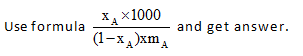QUESTION: 25

Molality : It is defined as the moles of the solute present in 1kg of the solvent. It is denoted by 'm'.Let WA grams of the solute of molecular mass mA be present in WB grams of the solvent, thenRelation between mole fraction and Molality :Q.

The mole fraction of the solute in the 12 molal solution of Na2CO3 is :

Solution: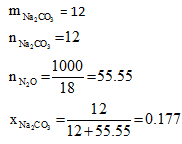QUESTION: 26

Molality : It is defined as the moles of the solute present in 1kg of the solvent. It is denoted by 'm'.Let WA grams of the solute of molecular mass mA be present in WB grams of the solvent, thenRelation between mole fraction and Molality :Q.

What is the quantity of water that should be added to 16 gm, methanol to make the mole fraction of methanol as 0.25 –

Solution: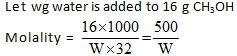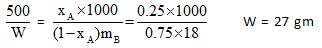QUESTION: 27

Molality : It is defined as the moles of the solute present in 1kg of the solvent. It is denoted by 'm'.Let WA grams of the solute of molecular mass mA be present in WB grams of the solvent, thenRelation between mole fraction and Molality :Q.

A 300 gm, 30% (wlw) NaOH solution is mixed with 500 gm 40% (wow) NaOH solution. What is % (wlw) NaOH. if density of final solution is 2 gm/ml.

Solution: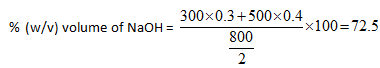QUESTION: 28

Molality : It is defined as the moles of the solute present in 1kg of the solvent. It is denoted by 'm'.Let WA grams of the solute of molecular mass mA be present in WB grams of the solvent, thenRelation between mole fraction and Molality :Q.

What is the molality of final solution obtained in the above problem

Solution: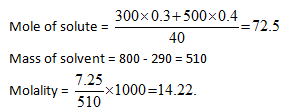QUESTION: 29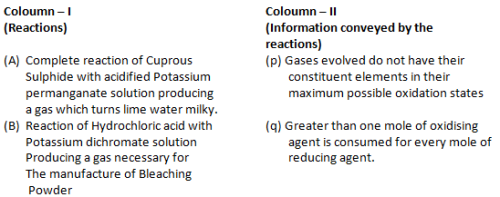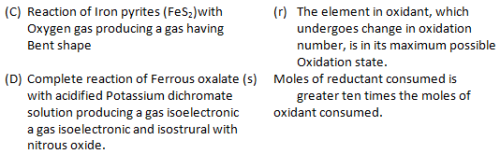Solution:

(A) 5Cu2S + 8 KMnO4  Cu2+ + SO2 + Mn2 +

vf = 8           vf = 5

(B) K2Cr2O7 + 14HCl  2KCl + 2 CrCl3 + 3Cl2+ 7H2O

(C) 4FeS2 + 11O2  2Fe2O3 + 8SO2(D) 6FeC2O4 + 3K2Cr2O7  Cr3 + + Fe3 + + CO2

Vf =3     Vf = 6

QUESTION: 30

Match the reacting mixture in coloumn-I with the reagent in coloumn – Il

Column – I                        Coloumn – Il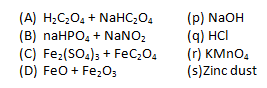Solution: